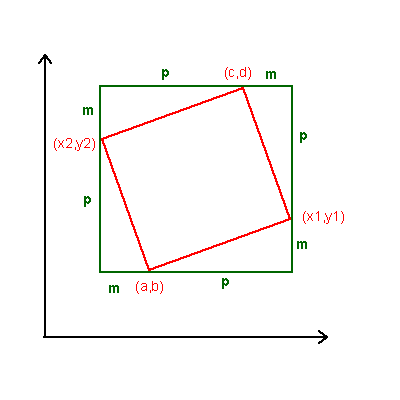#### You may also like### Diophantine N-tuples

Can you explain why a sequence of operations always gives you perfect squares?### DOTS Division

Take any pair of two digit numbers x=ab and y=cd where, without loss of generality, ab > cd . Form two 4 digit numbers r=abcd and s=cdab and calculate: {r^2 - s^2} /{x^2 - y^2}.### Sixational

The nth term of a sequence is given by the formula n^3 + 11n . Find the first four terms of the sequence given by this formula and the first term of the sequence which is bigger than one million. Prove that all terms of the sequence are divisible by 6.

# A Tilted Square

##### Age 14 to 16Challenge Level

Much of this solution uses the work of Andrew I and Elizabeth F. Well done.

For the square with opposite vertices of co-ordinates (5, 3) and (5, 7) respectively, you can obtain the other vertices (7, 5) and (3, 5) by creating 4 right-angled congruent triangles. The resulting figure is a square because: it has all its sides congruent, and all its angles right angles.

For the opposite corners of co-ordinates (5, 3) and (6, 6), using the same method, you get (4, 5) and (7, 4).

Veryfying that the four point sets from the problem are squares:

A. (8, 3); (7, 8); (2, 7); (3, 2) - Yes
B. (3, 3); (7, 4); (8, 8); (4; 7) - No
C. (16, 19); (18; 22); (21, 20); (19, 17) - Yes
D. (4, 20); (21, 19); (20, 2); (3, 3) - Yes

For the general case, using the drawing from the figure.

Let the co-ordinates of one corner be (a, b), and of the opposite be (c, d). Note that (x1, y1) and (x2, y2) are the co-ordinates of the other two corners. The geometrical problem has unique solution, that is obtained in the following manner:Using the values m and p

 x2 = c - p (i) y2 = d - m (ii) x1 = a + p (iii) y1 = b + m (iv) c = a +p - m (v) d = b + p + m (vi)

(v) + (vi) gives
c + d = a + b + 2p
p = (c + d - a - b)/2

(v) - (vi) gives
c - d = a - b - 2m
m = (a - b + d - c)/2

Substituting these values for m and p iinto (i), (ii), (iii) and (iv) gives:

x1 = (a + c + d - b)/2
y1 = (a + b + d - c)/2
x2 = (a + b + c - d)/2
y2 = (b + c + d - a)/2